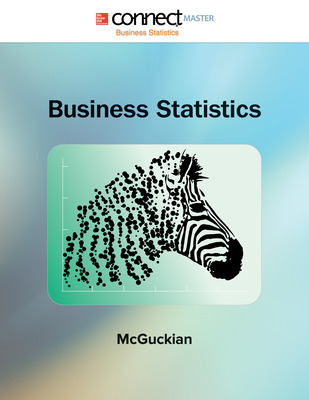# Connect Master for Business Statistics Online Access

1st Edition
By Dane McGuckian
ISBN10: 1259127680
ISBN13: 9781259127687
Product Details +

## Purchase Options

Students, we’re committed to providing you with high-value course solutions backed by great service and a team that cares about your success. See tabs below to explore options and pricing. Don't forget, we accept financial aid and scholarship funds in the form of credit or debit cards.

### Product

The estimated amount of time this product will be on the market is based on a number of factors, including faculty input to instructional design and the prior revision cycle and updates to academic research-which typically results in a revision cycle ranging from every two to four years for this product. Pricing subject to change at any time.

### Program Details

Module 1: Data and the Science of Statistics
• 1.1 The Discipline of Statistics
• 1.2 Types of Statistics
• 1.3 Sample Data vs. Population Data
• 1.4 Types of Data
• 1.5 Levels of Measurement

Module 2: Describing Attributes of a Data Set
• 2.1 Summarizing Qualitative Data
• 2.2 Summarizing Quantitative Data
• 2.3 Summation Notation
• 2.4 Measures of Center
• 2.5 Measures of Dispersion
• 2.6 Chebyshev's Theorem
• 2.7 The Empirical Rule
• 2.8 Relative Position
• 2.9 Box Plots and Symmetry

Module 3: A Survey of Probability
• 3.1 Basic Probability
• 3.2 Counting Techniques
• 3.3 Addition Rule of Probability
• 3.4 Conditional Rule of Probability
• 3.5 Multiplication Rule of Probability
• 3.6 Using Complements

Module 4: Discrete Random Variables
• 4.1 Probability Distributions for Discrete Random Variables
• 4.2 Expected Value, Variance, and Standard Deviation for Discrete Random Variables
• 4.3 The Binomial Probability Distribution
• 4.4 The Poisson Probability Distribution

Module 5: Continuous Random Variables

• 5.1 Continuous Random Variables
• 5.2 The Normal Distribution
• 5.3 Applications of the Normal Distribution
• 5.4 Normal Approximation to the Binomial Distribution

Module 6: Point Estimators and the Sampling Distribution of the Sample Mean
• 6.1 Point Estimators and Sampling Distributions
• 6.2 The Central Limit Theorem

Module 7: Interval Estimators
• 7.1 Interval Estimate of the Population Mean with a Known Population Standard Deviation
• 7.2 Sample Size Requirements for Estimating the Population Mean
• 7.3 Interval Estimate of the Population Mean with an Unknown Population Standard Deviation
• 7.4 Interval Estimate of the Population Proportion
• 7.5 Sample Size Requirements for Estimating the Population Proportion

Module 8: Hypothesis Testing
• 8.1 Testing Hypothesis about a Population Mean with a Known Population Standard Deviation
• 8.2 Testing Hypothesis about a Population Mean with an Unknown Population Standard Deviation
• 8.3 Testing Hypothesis about a Population Proportion

Module 9: Two Sample Procedures
• 9.1 Comparisons of Two Population Means with Known Population Standard Deviations Based on Independent Samples
• 9.2 Comparisons of Two Population Means with Unknown Population Standard Deviations Based on Independent Samples with Equal Variances
• 9.3 Comparisons of Two Population Means with Unknown and Assumed Unequal Population Variances Based on Independent Samples
• 9.4 Inferences about Mean Differences (Dependent Samples)
• 9.5 Comparisons of Two Population Proportions Based on Independent Sampling
• 9.6 Comparisons of Two Population Variances Based on Independent Samples

Module 10: Chi-Square Procedures
• 10.1 The Chi-Square Goodness-of-Fit Test
• 10.2 The Chi-Square Test of Independence

Module 11: Linear Models
• 11.1 ANOVA for Completely Randomized Design Experiments
• 11.2 The Coefficient of Correlation
• 11.3 Creating the Least Squares Equation
• 11.4 Making Inference about the Slope of the Regression Line
• 11.5 The Coefficient of Determination
• 11.6 Confidence and Prediction Intervals for Y